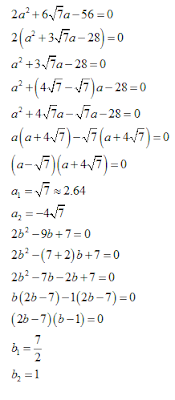# Quadratic Equations for SBI PO 2017: Part-1

1. In the following question two equations (A) and (B) given. You have to solve both:
a. 21a² - 20a -9=0
b. 7b² -23b +18=0

1. If a > b
2. If a<b
3. If a = b or C.N.E
4. If a > b
5. If a <
2. In the following question two equations (A) and (B) given. You have to solve both:
a. 48a² + 2a -1 = 0
b. 6b² - 11b -2 = 0

1. If a<b
2. If a b
3. If a > b
4. If a < b
5. If a = b or C.N.E

3. In the following question two equations (A) and (B) given. You have to solve both:
a.
9a² - 24a  + 143 = 0
b. 9b² + 50b - 91 = 0

1. If a >b
2. If a<b
3. If a > b
4. If a = b or C.N.E
5. If a < b

4. In the following question two equations (A) and (B) given. You have to solve both:
a. 36a² - 19a - 7 = 0
b. 12b² - 5b - 2 = 0

1. If a < b
2. If a = b or C.N.E
3. If a<b
4. If a > b
5. If a < b

5. In the following question two equations (A) and (B) given. You have to solve both:1. If a < b
2. If a>b
3. If a = b or C.N.E
4. If a < b
5.  If a > b

## Solutions:

#### 1. 5#### 2. 5#### 3. 3#### 4. 2#### 5. 3#### What's trending in BankExamsToday

Smart Prep Kit for Banking Exams by Ramandeep Singh - Download here